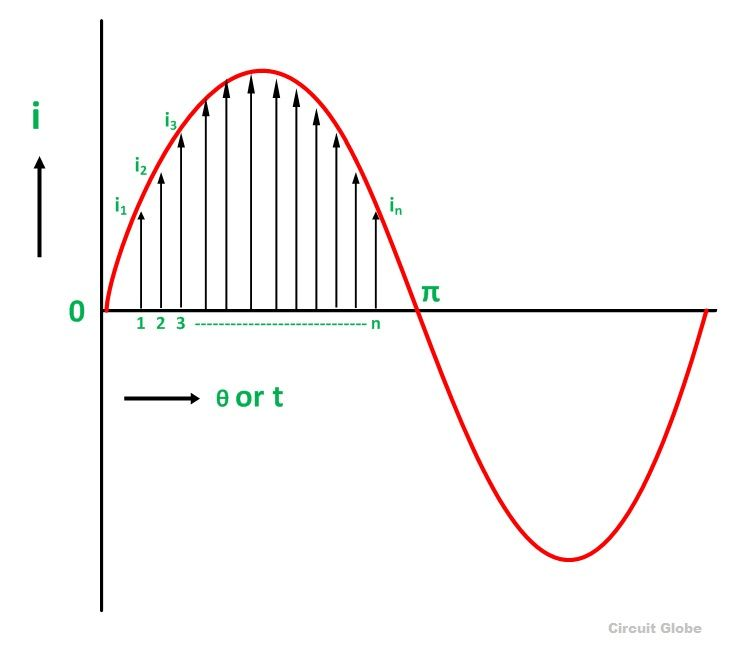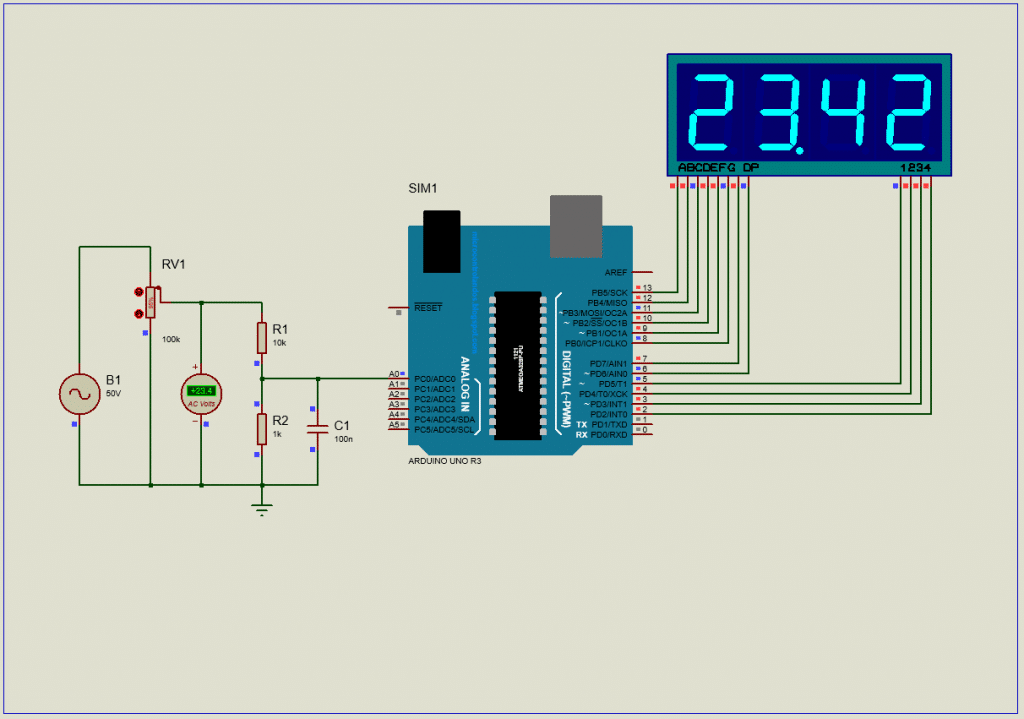# How to make an AC voltmeter using Arduino UNO and 4×1 multiplexed Seven Segment display

In this article, we will learn to make an AC voltmeter using Arduino UNO and 4×1 seven segment display. If you learn this project clearly, you’ll be able to measure any AC voltage of your home. I hope you’ll make your own AC voltmeter yourself now.

Disclaimer: Electricity is always dangerous. Skill required to work with electricity. Do work with your own risk. Author will not be responsible for any misuse or harmful act. This website contents is also copyright protected. Anything copied and directly posted in your website and claiming it as yours is prohibited and nonsensical. Author published all the articles as opensource to help you making your project and learning purpose only. Learn and make one for yourself. If need any help feel free to ask the author. Author will be helpful to you. Thanks.

Table of Contents

## What is the difference in AC voltage measurement?

Like the DC voltmeter [check other article on DC voltmeter with Arduino] input voltage is not fixed in a point, it is alternating 50 times or 60 times per second. Whether you are in 50Hz or 60Hz supply area, the measuring concept is same.AC signal

If you measure like the DC voltmeter, you’ll get a fluctuating reading. That is why, we need to follow a technique.

## AC voltage measurement technique:

As the signal is alternating, so we can not measure with average reading only. But if we measure the peak points and calculate an average reading of some peak values, we can get the exact peak point result.

Now, we know how to get the rms value from the peak value.

Vrms = Vpeak x 0.707

To find the peak point, we can use Arduino peak point function. Also we can do our own coding to find the peak point.

## Circuit diagram:Circuit diagram

## Arduino Coding:

```#include <TimerOne.h>
const char segment_pins[] = {13, 12, 11, 10, 9, 8, 7, 6}; //segments a to g
const char digit_pins[] = {5, 4, 3, 2}; // 4digits
const int digit_number = 4;//for 4 digit segment
byte dot_position;//set dot position from right side.
bool dot_point = true; // having dot or not, true = have dot, false = no dot.

const int segment_array = // for common cathode segment only
{
{HIGH, HIGH, HIGH, HIGH, HIGH, HIGH, LOW, LOW}, //0
{LOW, HIGH, HIGH, LOW, LOW, LOW, LOW, LOW},     //1
{HIGH, HIGH, LOW, HIGH, HIGH, LOW, HIGH, LOW},  //2
{HIGH, HIGH, HIGH, HIGH, LOW, LOW, HIGH, LOW},  //3
{LOW, HIGH, HIGH, LOW, LOW, HIGH, HIGH, LOW},   //4
{HIGH, LOW, HIGH, HIGH, LOW, HIGH, HIGH, LOW},  //5
{HIGH, LOW, HIGH, HIGH, HIGH, HIGH, HIGH, LOW}, //6
{HIGH, HIGH, HIGH, LOW, LOW, LOW, LOW, LOW},    //7
{HIGH, HIGH, HIGH, HIGH, HIGH, HIGH, HIGH, LOW},//8
{HIGH, HIGH, HIGH, HIGH, LOW, HIGH, HIGH, LOW}, //9
};

void int_sev_segment()
{
for (int k = 0; k < 8; k++)
{
pinMode(segment_pins[k], OUTPUT);//I/O settings
}
for (int k = 0; k < digit_number; k++)
{
pinMode(digit_pins[k], OUTPUT);//I/O settings
}
for (int k = 0; k < 8; k++)
{
digitalWrite(segment_pins[k], LOW);//keep low
}
for (int k = 0; k < digit_number; k++)
{
digitalWrite(digit_pins[k], HIGH);//keep high = disable segments (CC)
}
Timer1.initialize(5000);//5000us = 5ms
Timer1.attachInterrupt(display_segment);
}

char digits;
void display_7segment(int number, byte dot)
{
digits = number / 1000u;//extract 1000th digit
digits = (number / 100u) % 10u;//extract 100th digit
digits = (number / 10u) % 10u;//extract 10th digit
digits = (number / 1u) % 10u;//extract 1st digit
dot_position = dot;
}

int digit_position = 0;
void display_segment(void) // called periodically using timer interrupt
{
for (int k = 0; k < digit_number; k++)
{
digitalWrite(digit_pins[k], HIGH);//reset digit pins
}
if (digit_position > 3)digit_position = 0;

for (int k = 0; k < 8; k++) //print the a to g segment pins
{
digitalWrite(segment_pins[k], segment_array[digits[digit_position]][k]);
if (digit_position == dot_position && dot_point == true)
{
digitalWrite(segment_pins, HIGH);//print dot point
}
}

if (digit_position == 3)
{
digitalWrite(digit_pins, LOW);
digitalWrite(digit_pins, HIGH);
digitalWrite(digit_pins, HIGH);
digitalWrite(digit_pins, HIGH);
}
else if (digit_position == 2)
{
digitalWrite(digit_pins, HIGH);
digitalWrite(digit_pins, LOW);
digitalWrite(digit_pins, HIGH);
digitalWrite(digit_pins, HIGH);
}
else if (digit_position == 1)
{
digitalWrite(digit_pins, HIGH);
digitalWrite(digit_pins, HIGH);
digitalWrite(digit_pins, LOW);
digitalWrite(digit_pins, HIGH);
}
else if (digit_position == 0)
{
digitalWrite(digit_pins, HIGH);
digitalWrite(digit_pins, HIGH);
digitalWrite(digit_pins, HIGH);
digitalWrite(digit_pins, LOW);
}
digit_position++;
}

long ac_voltage = 0;
unsigned int temp, maxpoint;
void setup()
{
int_sev_segment();//initialize seven segment program
}

void loop()
{
ac_voltage = 0; //clear previous result
temp = 0;
maxpoint = 0;
for (int i = 0; i < 20; i++)
{
for(int m=0;m<1000;m++)
{
if(temp = analogRead(A0);temp>maxpoint)
{
maxpoint = temp;
}
}
ac_voltage += maxpoint*3.79659;
}
ac_voltage /= 20; //get average value

display_7segment(ac_voltage, 2); //display value of cnt, dot in position 1 from right side

}

// end```

Like the previous post on DC voltmeter with Arduino UNO, here the only change is in AC voltage measurement function.

```  ac_voltage = 0; //clear previous result
temp = 0;
maxpoint = 0;
for (int i = 0; i < 20; i++)
{
for(int m=0;m<1000;m++)
{
if(temp = analogRead(A0);temp>maxpoint)
{
maxpoint = temp;
}
}
ac_voltage += maxpoint*3.79659;
}
ac_voltage /= 20; //get average value```

Here, we are using our own function to find the peak point. Then we calculated the rms value from that peak value. And finally we are taking an average value of 20 readings.

```ac_voltage = 0; //clear previous result
temp = 0;
maxpoint = 0;
for (int i = 0; i < 10; i++)
{
for(int m=0;m<500;m++)
{
if(temp = analogRead(A0);temp>maxpoint)
{
maxpoint = temp;
}
}
ac_voltage += maxpoint*3.79659;
}
ac_voltage /= 10; //get average value```

Here, taking fewer sample will make the meter more responsive.

## Conclusion:

In this project, you learned how to make an AC voltmeter that can measure low voltage AC with Arduino and Op-Amp circuit. The result is displayed in multiplexed Seven segment display. We tested the voltmeter in simulation. This project is now ready for practical use.

Kindly select voltage divider resistors carefully to measure higher voltage. Proper selection can increase the resolution of our voltmeter.

But keep in mind that electricity is always dangerous. Do work on your own risk.

I hope, you enjoyed this project and now you can make one for yourself. If you are stuck in middle and need help, just ask. I’ll try my best to help you. Thank you, Enjoy!

#### MKDas

Mithun K. Das; B.Sc. in EEE from KUET. Blog: https://labprojectsbd.com